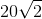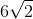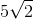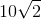# High School Math : How to find the perimeter of a square

## Example Questions

### Example Question #1 : How To Find The Perimeter Of A Square

A circle with a radius 2 in is inscribed in a square. What is the perimeter of the square?

16 in

24 in

28 in

12 in

32 in

16 in

Explanation:

To inscribe means to draw inside a figure so as to touch in as many places as possible without overlapping. The circle is inside the square such that the diameter of the circle is the same as the side of the square, so the side is actually 4 in.  The perimeter of the square = 4s = 4 * 4 = 16 in.

### Example Question #11 : Quadrilaterals

Square X has 3 times the area of Square Y.  If the perimeter of Square Y is 24 ft, what is the area of Square X, in sq ft?

108

112

144

72

54

108

Explanation:

Find the area of Square Y, then calculate the area of Square X.

If the perimeter of Square Y is 24, then each side is 24/4, or 6.

A = 6 * 6 = 36 sq ft, for Square Y

If Square X has 3 times the area, then 3 * 36 = 108 sq ft.

### Example Question #1 : How To Find The Perimeter Of A Square

A square has an area of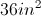.  If the side of the square is reduced by a factor of two, what is the perimeter of the new square?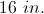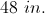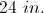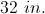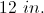Explanation:

The area of the given square is given by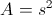so the side must be 6 in.  The side is reduced by a factor of two, so the new side is 3 in.  The perimeter of the new square is given by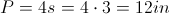.

### Example Question #1 : How To Find The Perimeter Of A Square

A square garden has an area of 64 square feet. If you add 3 feet to each side, what is the new perimeter of the garden?

44

121

32

20

25

44

Explanation:

By finding the square root of the area of the garden, you find the length of one side, which is 8. We add 3 feet to this, giving us 11, then multiply this by 4 to get 44 feet for the perimeter.

### Example Question #5 : How To Find The Perimeter Of A Square

The area of the shaded region of a square is 18. What is the perimeter of the square?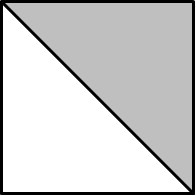20

36

28

24

24

Explanation:

The area of the shaded region, which covers half of the square is 18 meaning that the total area of the square is 18 x 2, or 36. The area of a square is equal to the length of one side squared. Since the square root of 36 is 6, the length of 1 side is 6. The perimeter is the length of 1 side times 4 or 6 x 4.

### Example Question #6 : How To Find The Perimeter Of A Square

The area of a square is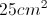.  If the square is enlarged by a factor of 2, what is the perimeter of the new square?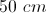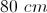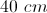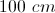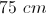Explanation:

The area of a square is given byso we know the side is 5 cm.  Enlarging by a factor of two makes the new side 10 cm.  The perimeter is given by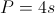, so the perimeter of the new square is 40 cm.

### Example Question #4 : How To Find The Perimeter Of A Square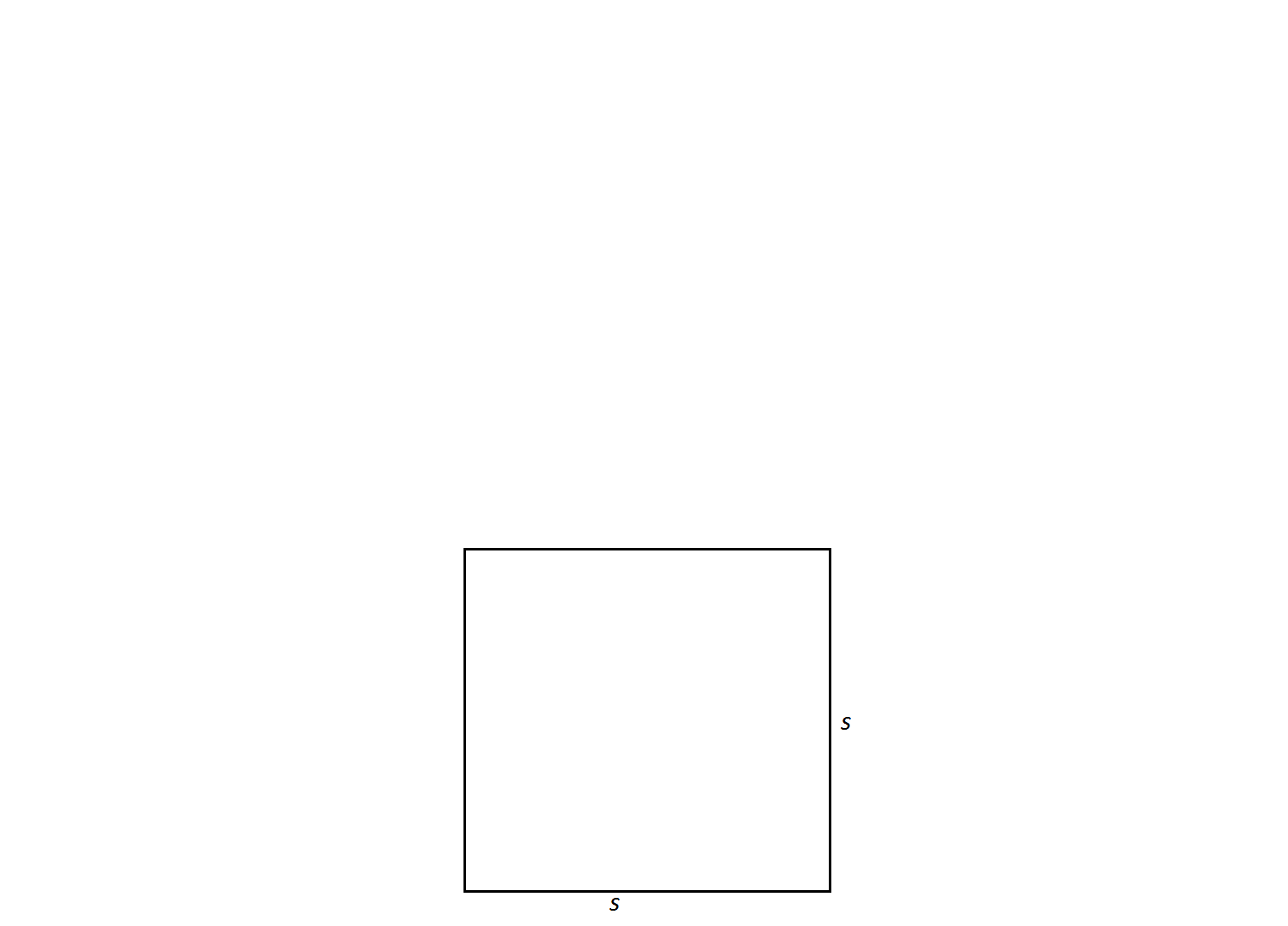A square has a side length of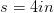. Find its perimeter.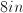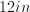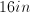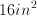Not enough information to solveExplanation:

In order to find the perimeter of a square, one must add up all of its side lengths. The side lengths of a square are all equal; therefore, the formula for the perimeter of a square is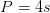.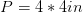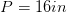Remember perimeter should be expressed in single units not units squared. Thus,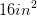is an incorrect answer.

### Example Question #1 : How To Find The Perimeter Of A Square

The diagonal of a square has a length of 10 inches. What is the perimeter of the square in inches squared?